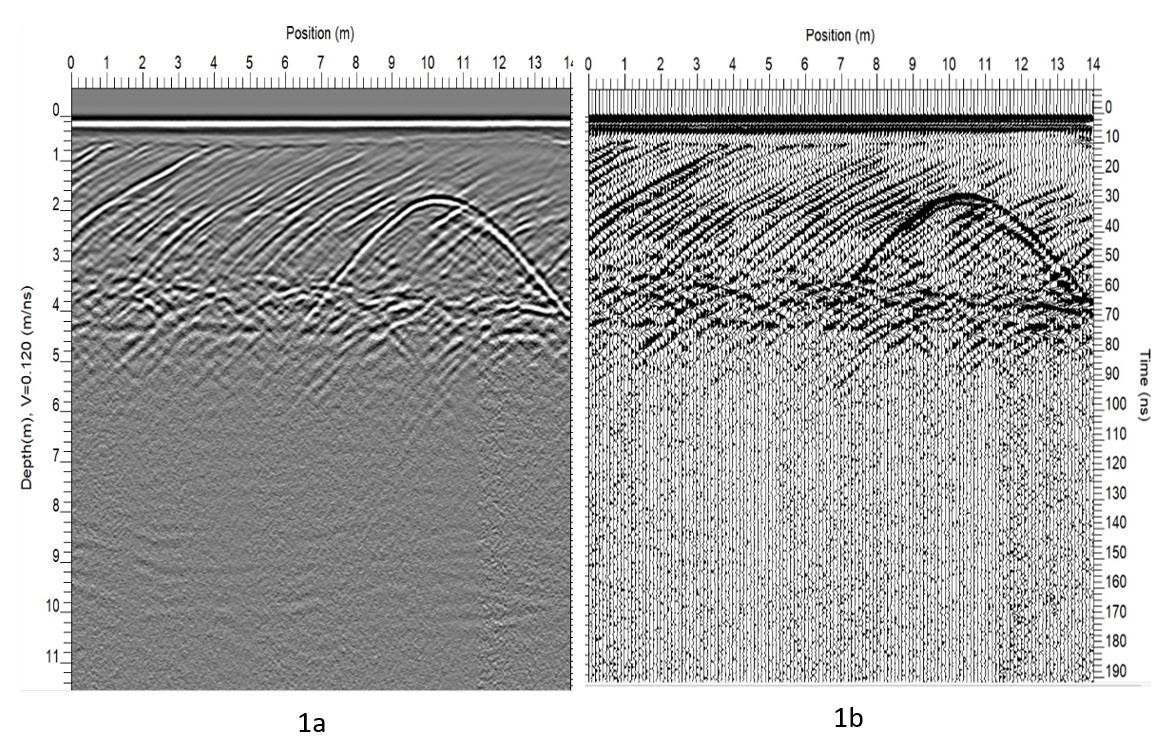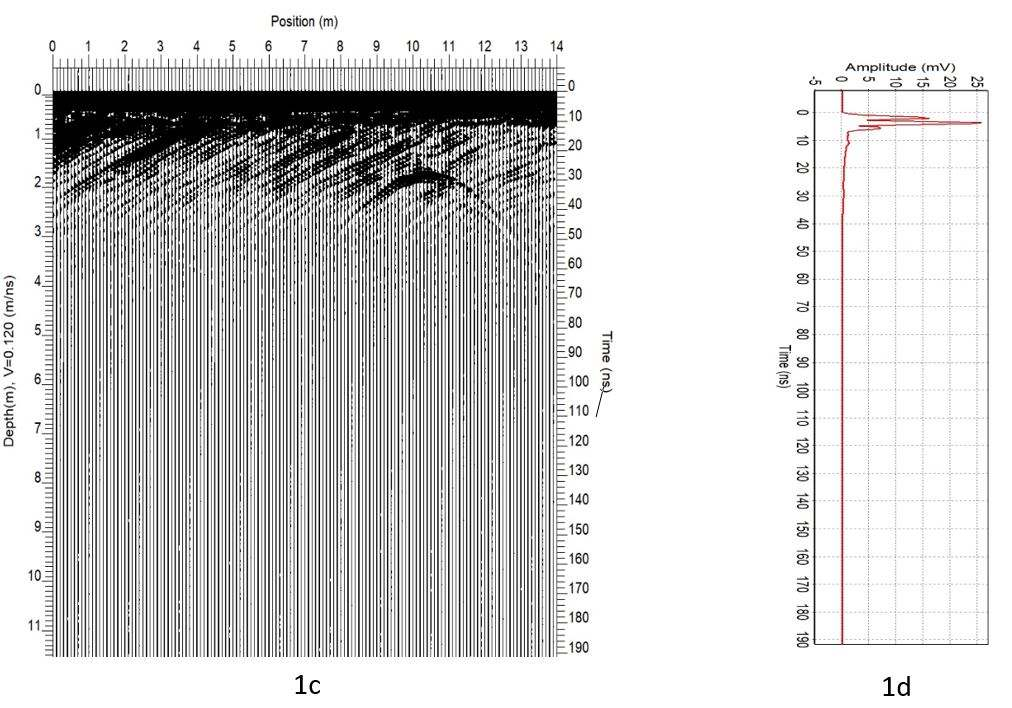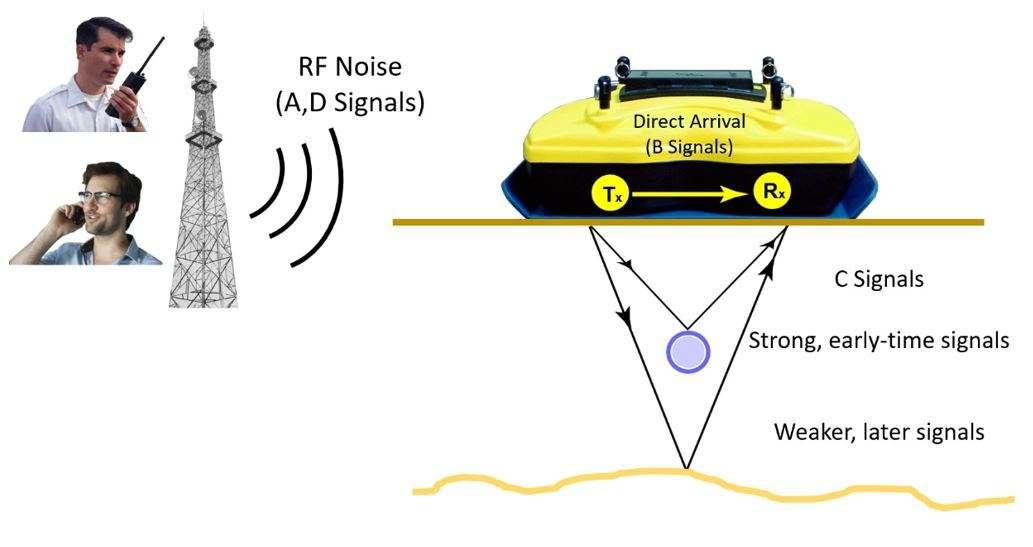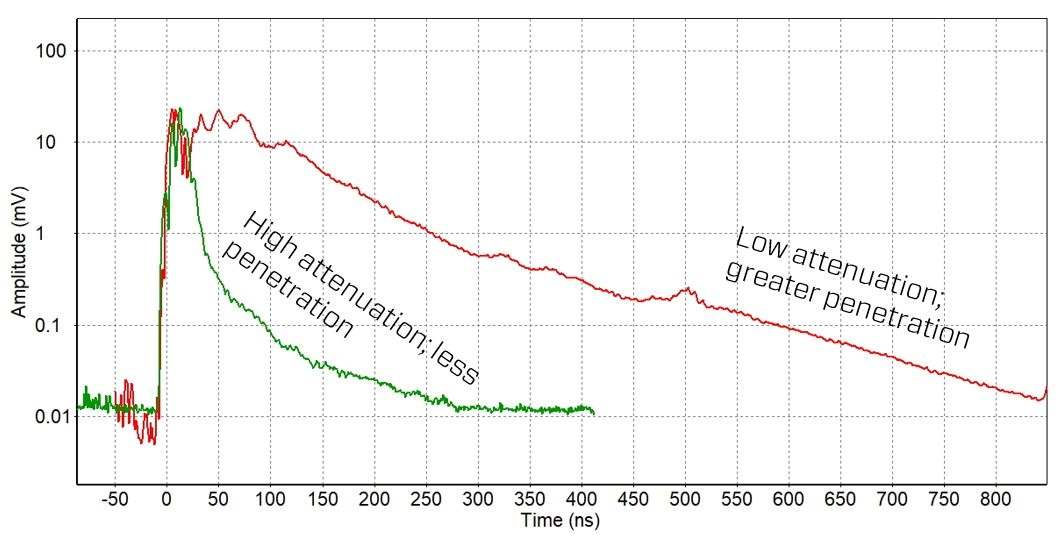The Power of Average Trace Amplitude (ATA) PlotsX

# The Power of Average Trace Amplitude (ATA) Plots

P

lotting the average of all the traces in a GPR cross-section shows the response character versus time and provides the user with insights and key understandings of the nature of the data.

GPR lines are commonly displayed in varying colors based on signal amplitude (Figure 1a).  However, the best way to show trace data is by plotting the GPR data as a wiggle trace (Figure 1b).  A wiggle trace plot depicts the amplitudes of the signal as deflections from zero amplitude.Figure 1:
GPR line plotted in variable color (gray shades) traces (a) and wiggle trace (b). To create an ATA plot, all the traces are rectified (c) and then averaged to a single trace (d).

To generate an ATA plot, all the traces from a GPR line are rectified (also called absolute valued) to remove the negative signal amplitudes and show all the signals as positive amplitudes (Figure 1c).  Then, the rectified traces are averaged to a single trace (Figure 1d).  The averaging of rectified data means that the noise and signals are all included in the resulting average.

ATA plots are usually plotted with the average trace on its side (Figure 2); time in the X direction and amplitude in the Y direction.  Also, since there is such a large dynamic range in the GPR signal amplitudes, the average trace amplitude plot is usually plotted with a logarithmic amplitude scale.

1. The plot can be used for assessing and quantifying random noise and the depth of penetration of the GPR signals
2. Flat lying reflectors are emphasized; reflectors that dip or vary in depth are averaged out
3. Coherent system noise which is time-invariant will show up in the ATA plot and can be diagnosed as such
4. The plot shows if the GPR signals are being clipped off
5. The decay curve of the amplitude with time is a good measure of ground attenuation
6. The ATA amplitude decrease provides a guide to the appropriate time gain function to be applied to data

In this article, we focus on how to use the Average Trace-Amplitude (ATA) plot for a and e.Figure 2:
Average rectified trace plotted in a logarithmic scale. A and D = background RF noise, B = the transmit pulse and C = attenuating signals. The “Depth of GPR Penetration” is the depth that GPR signals have attenuated down to the RF noise floor.

The GPR receiver begins recording before the GPR transmitter fires, resulting in only background radio frequency (RF) noise in the data (A in Figures 2 and 3).

After the GPR transmitter fires, the highest amplitude signal in an ATA plot is usually the direct arrival of the GPR transmit pulse at the receiver; this signal travels through air at the speed of light (B in Figures 2 and 3). This is called the “direct air wave”.

Following the direct pulse, GPR signals arriving at the receiver are weaker, as they have been attenuated after travelling through the subsurface. The further the GPR signals travel in the ground, the weaker they are and the later they arrive (C in Figures 2 and 3).

After all the GPR signals have attenuated, the receiver again records the background RF noise (D in Figures 2 and 3).Figure 3:
Illustration of the types of signals received by the GPR

The depth at which GPR signals are the same amplitude as the background noise (so they can no longer be differentiated) is defined as the GPR “Depth of Penetration”; this depth of penetration varies based on the electrical properties of the material (Figure 5).

## Background RF Noise

Before the GPR transmitter fires, the GPR receiver is recording other radio frequency emitters in its bandwidth (A in Figure 2). In the example in Figure 2, the background noise floor is approximately 0.004 millivolts.

Examples of ATA plots of 100 MHz data with background random noise varying from 0.03 to 2 mV (66x stronger) are shown in Figure 4. Background noise can vary tremendously depending on the RF environment – areas, usually urban areas, with many strong radio transmitters can generate signals 1000 times stronger than other, more remote or rural areas with few RF emitters.
High background noise reduces the depth of GPR penetration.Figure 4:
ATA plots showing varying levels of background radio frequency noise.

The ATA plots show that GPR amplitudes decay with time. Figure 5 shows extreme attenuation curves of 100 MHz antennas. The red line slopes very gradually, indicating low attenuation and deep GPR signal penetration. In fact, at 850 ns (about 45 meters), the signal has still not attenuated down to the noise floor; this means that the operator would have seen deeper if they set a longer time window. The green line shows higher attenuation, with the GPR signal rapidly falling into the background noise floor, indicating limited GPR signal penetration at this site.Figure 5:
Low (red line) and high (green line) attenuation curves.

We’ve only just scratched the surface of the value of ATA plots in this TIPS article. In addition to background noise and depth of penetration analysis, ATA plots can also help identify coherent system noise and air waves. Stay tuned for future articles on this topic.

When used in a controlled manner, the ATA method is extremely powerful and should be a methodology used by all professional GPR analysts. ATA plots are available in the Processing module of the EKKO_Project™ software.

﻿

﻿